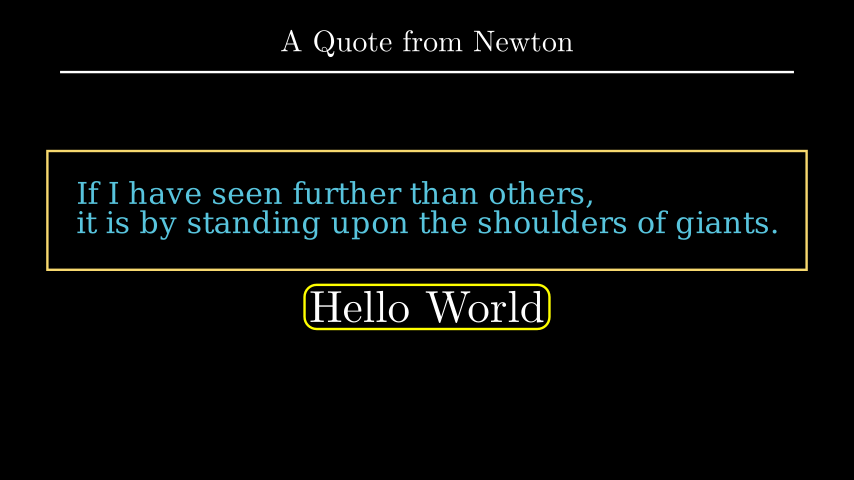# SurroundingRectangle#

Qualified name: `manim.mobject.geometry.shape\_matchers.SurroundingRectangle`

class SurroundingRectangle(mobject, color=ManimColor('#FFFF00'), buff=0.1, corner_radius=0.0, **kwargs)[source]#

A rectangle surrounding a `Mobject`

Examples

Example: SurroundingRectExample```from manim import *

class SurroundingRectExample(Scene):
def construct(self):
title = Title("A Quote from Newton")
quote = Text(
"If I have seen further than others, \n"
"it is by standing upon the shoulders of giants.",
color=BLUE,
).scale(0.75)
box = SurroundingRectangle(quote, color=YELLOW, buff=MED_LARGE_BUFF)

t2 = Tex(r"Hello World").scale(1.5)
mobjects = VGroup(VGroup(box, quote), VGroup(t2, box2)).arrange(DOWN)
```
```class SurroundingRectExample(Scene):
def construct(self):
title = Title("A Quote from Newton")
quote = Text(
"If I have seen further than others, \n"
"it is by standing upon the shoulders of giants.",
color=BLUE,
).scale(0.75)
box = SurroundingRectangle(quote, color=YELLOW, buff=MED_LARGE_BUFF)

t2 = Tex(r"Hello World").scale(1.5)
mobjects = VGroup(VGroup(box, quote), VGroup(t2, box2)).arrange(DOWN)

```

Methods

Attributes

 `animate` Used to animate the application of any method of `self`. `animation_overrides` `color` `depth` The depth of the mobject. `fill_color` If there are multiple colors (for gradient) this returns the first one `height` The height of the mobject. `n_points_per_curve` `sheen_factor` `stroke_color` `width` The width of the mobject.
Parameters:
• mobject (Mobject) –

• color (ParsableManimColor) –

• buff (float) –

Initialize self. See help(type(self)) for accurate signature.

Parameters:
• mobject (Mobject) –

• color (Union[ManimColor, int, str, Tuple[int, int, int], Tuple[float, float, float], Tuple[int, int, int, int], Tuple[float, float, float, float], ndarray[Any, dtype[int64]], ndarray[Any, dtype[float64]]]) –

• buff (float) –